Search QuantityWare

# BCG & BCP Configuration FAQs

Answers to common questions regarding configuring bulk calculations for LPG / NGL products on your systems

### What is the difference between LPG and NGL?

From a BCS measurement standard point of view (considering the basic thermodynamical properties of temperature, density, pressure / vapor pressure) , LPG and NGL (high vapor pressure) are covered by the same group of supported BCS measurement standards*. Thus, either a BCG or a BCP license is required for quantity conversions of LPG and NGL, but not both.
From a business point of view, cosidering the financial values, there are considerable differences:

• In a nutshell, LPG are – from the business point of view (in many countries) –

### Can we combine an LPG vapor space model with any product, not just classic LPG products?

Yes.
The QuantityWare BCS template contains many examples for GPA 8217 and ISO 6578 in combination with one of the three available LPG vapor models. However, any of these three models can be combined with any other meaningful CTL standard – this makes sense for products which have a vapor pressure above standard atmospheric pressure at reference conditions – , e.g. national standards for LPG or bulk chemical products.

In order to utilize an LPG vapor space model for other CTL standards, MQCI function /QTYW/MQCI_SET_CTPL_TANK_VAPOR must be included into the configuration of your conversion group.

### How do I use LPG vapor space calculations to determine the amount of LPG transferred?

When transferring LPG, we need to accurately measure the amount transferred. To do this, we make use of LPG vapor space calculations.

The video below describes the details of LPG storage, along with details of how to perform static LPG vapor space calculations using both transferred mass calculation and movement analysis methods, via QuantityWare’s Bulk Calculations Solution (BCS):

Vimeo Video

### We utilize an LPG conversion group with vapor space calculations. Why is the vapor mass not considered for volume UoM?

Vapor space calculations are defined in measurement standard ISO 6578. The calculation simply adds the vapor mass (and vapor energy or weight) to the appropriate liquid mass, energy and weight quantity (in any given UoM).
Volume quantity values are not updated with the liquid volume quantity value equivalent to the vapor mass and thus do contain the liquid product quantity value only (in any given volume UoM).
Therefore, it is important that the base UoM in the SAP material master is either a mass, weight or energy UoM.

Read the working paper “LPG vapor space calculations”

### How are the two reading group indicators defined, that control the LPG vapor space calculations?

LPG quantity conversions support two vapor space scenarios. For each scenario, one indicator is relevant.

Scenario A: You perform an opening and a closing dip measurement (e.g. for a loading process shore-to-ship) to determine the transferred mass of LPG (e.g. by using SAP Tank Management and implementing your delta calculation logic in a SAP Tank Management BAdI):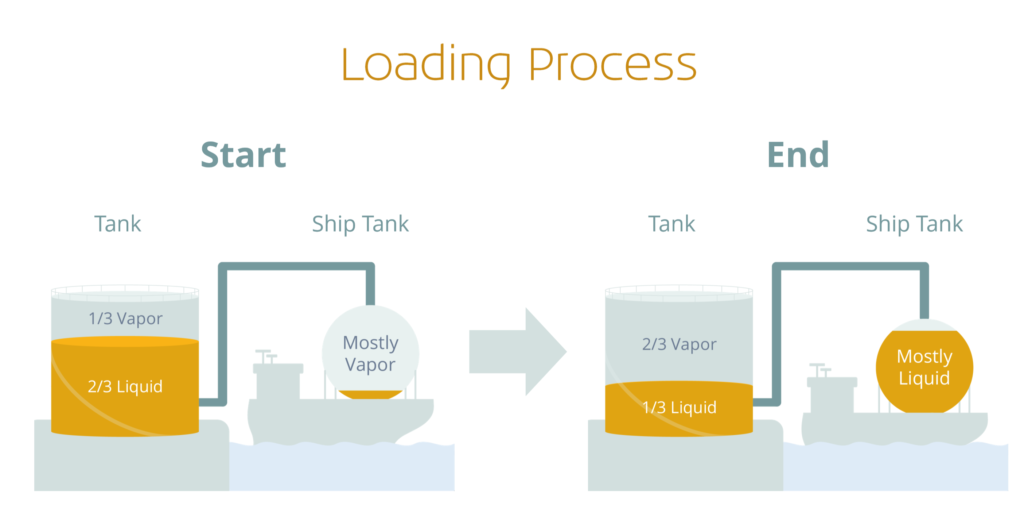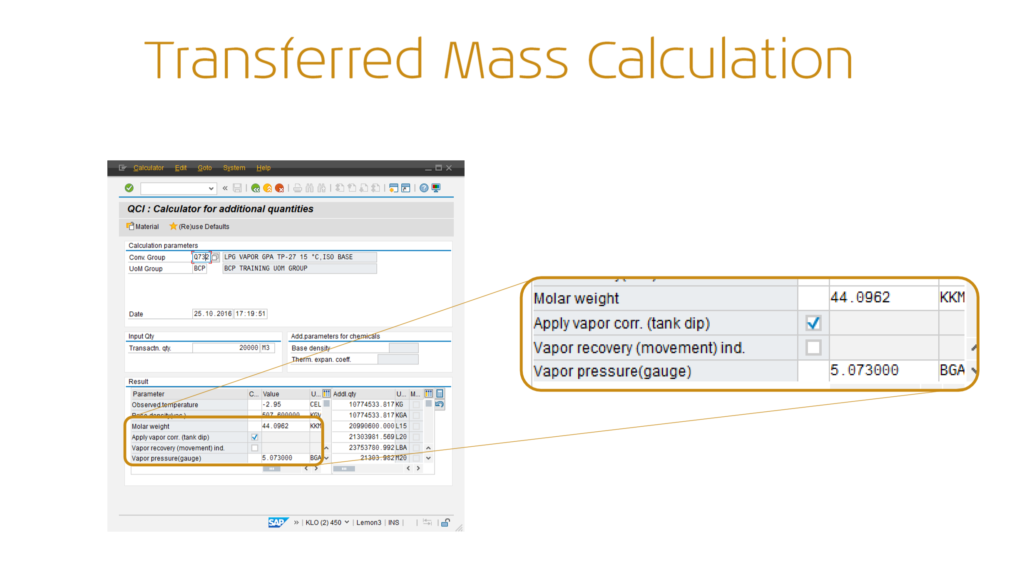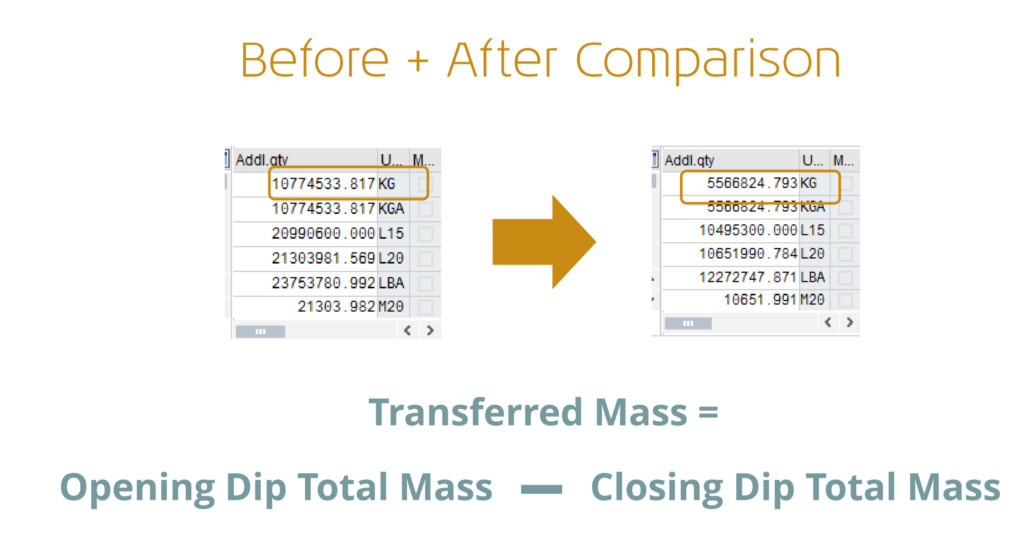Summary: In this scenario, you perform two calculations (opening and closing dip calculation) with the “Apply vapor corr. (tank dip)” indicator set. The transferred mass is calculated by subtraction.

### Which chemical components can I add to an ISO 6578 LPG reading group?

ISO 6578 provides two density calculation methods for LPG density calculations, based on the known chemical composition of the LPG.  Very large factors may have to be applied for the correction of observed density to density at standard temperature, e.g. a correction for the effect of a temperature difference of 60 °C may be necessary for refrigerated propane. Provided that the LPG does not contain more than 20 % of unsaturated hydrocarbons, the correction tables introduced in ISO 91 shall be used for volume corrections. Thus, ISO 6578 is intended for LPG products that contain more than 20 % of unsaturated hydrocarbons (e.g.

### Our LPG conversion group does not allow entry of a test temperature to correct the LPG density to base. How can we solve this requirement?

Many of the MQCI LPG Q7** template conversion groups (as well as many crude oil and product Q2**/ Q1** and some linear DCF conversion groups, e.g. Q0A9)  are configured such that the product base density (in vacuo or for DCF calculaitons – in air) can be entered directly without a need to enter a second so-called test temperature to correct a test density to base.  This is described in more detail in the BCP Reference Manual, Chapter 6.10.

Example: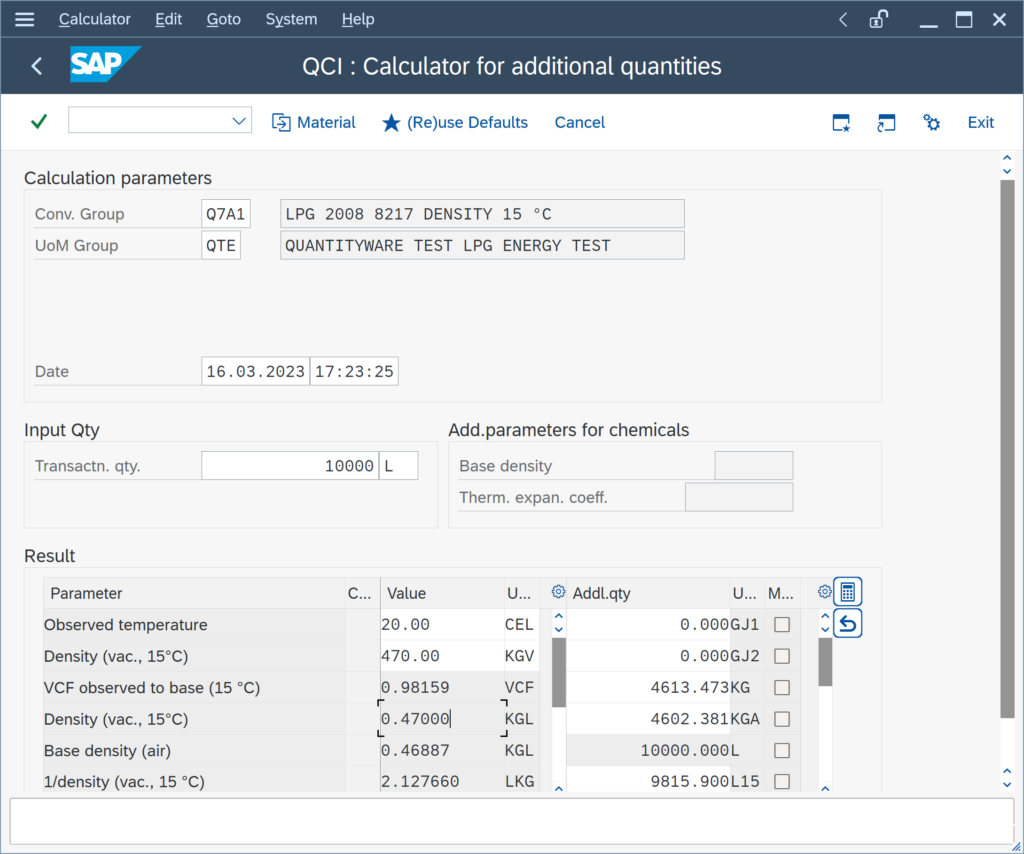If required, you can extend your LPG reading group and add the test temperature (technical name OBSTSTMETT) and then also change the name of the input parameter OBSTSTDENS from base density to e.g.

### We enter pressure values for gas calculations. Are these values absolute or gauge pressure values?

QuantityWare BCS implementations for LPG/NGL, LNG and natural gas support both the data entry of absolute and gauge pressure values. The conversion group configuration can be made to support entry of either gauge or absolute pressure values – by adding or removing dedicated MQCI pressure calculation functions to the conversion group function module sequence.

If you execute the Conversion Group Check, a message is issued that informs if pressure values (input data) are considered as absolute or gauge values.

Example 1: Template Conversion Group Q741: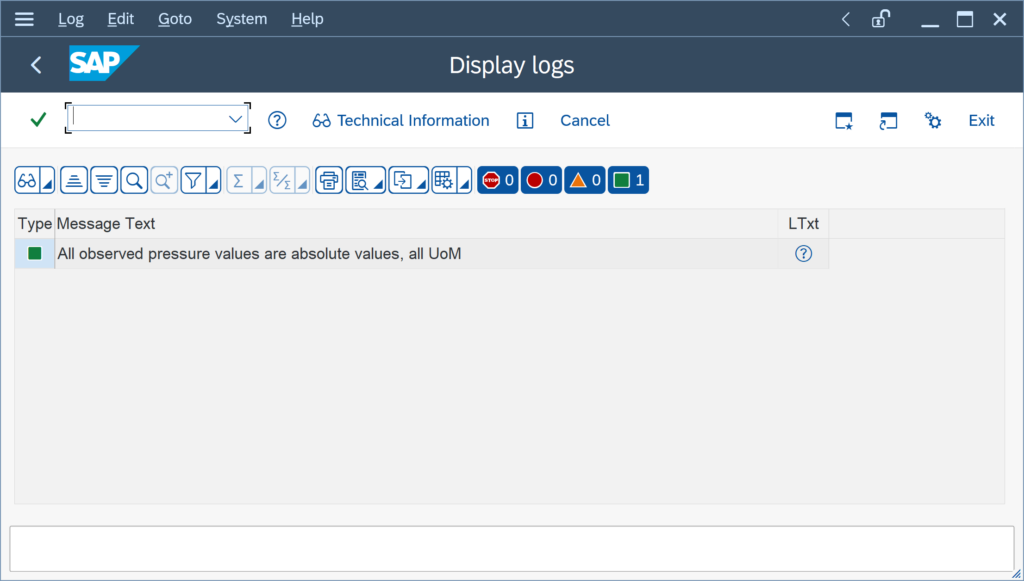Example 2: Template Conversion Group Q154: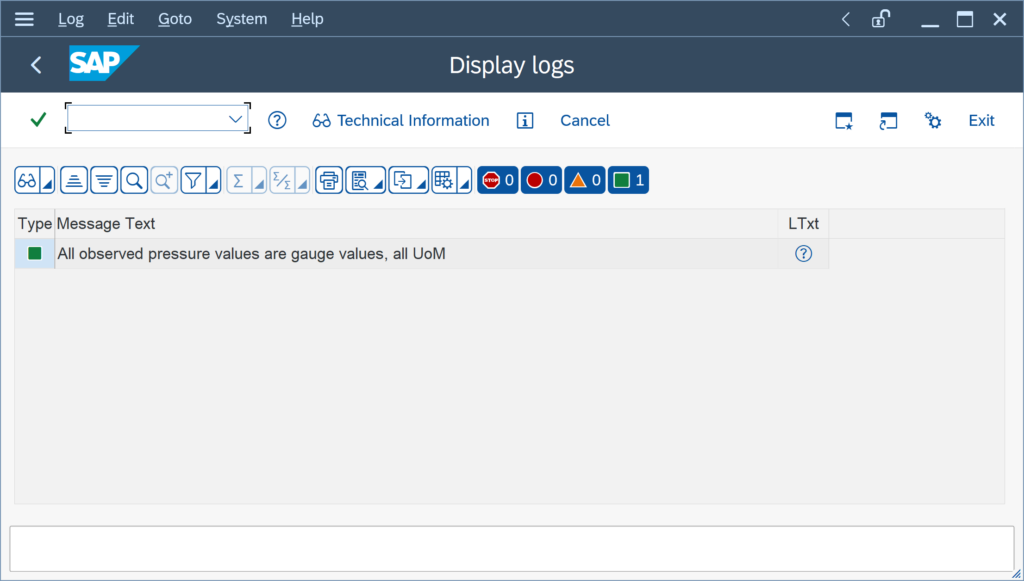### My rounding settings for the density values for an MQCI conversion group are not considered for the calculation. How can I fix this?

You have copied e.g. template conversion group Q1A1 to Z1A1. For the density UoM PPG (pounds per gallon – lb/ugl) you have maintained a rounding to 4 decimals; business requires that the rounded value is utilized for the calculation of masses.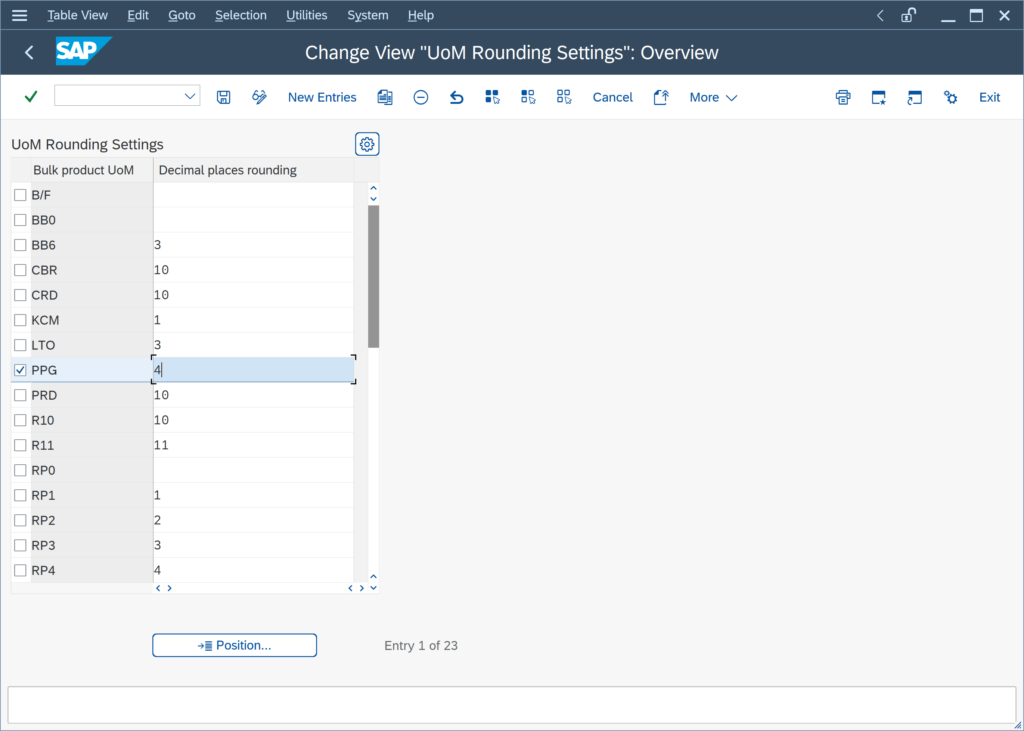A simple test calculation with Q1A1 shows the issue: The expected value for UoM LB is 620 540.00 LB; however, the value is calculated using the non-rounded density (while the rounded value is displayed):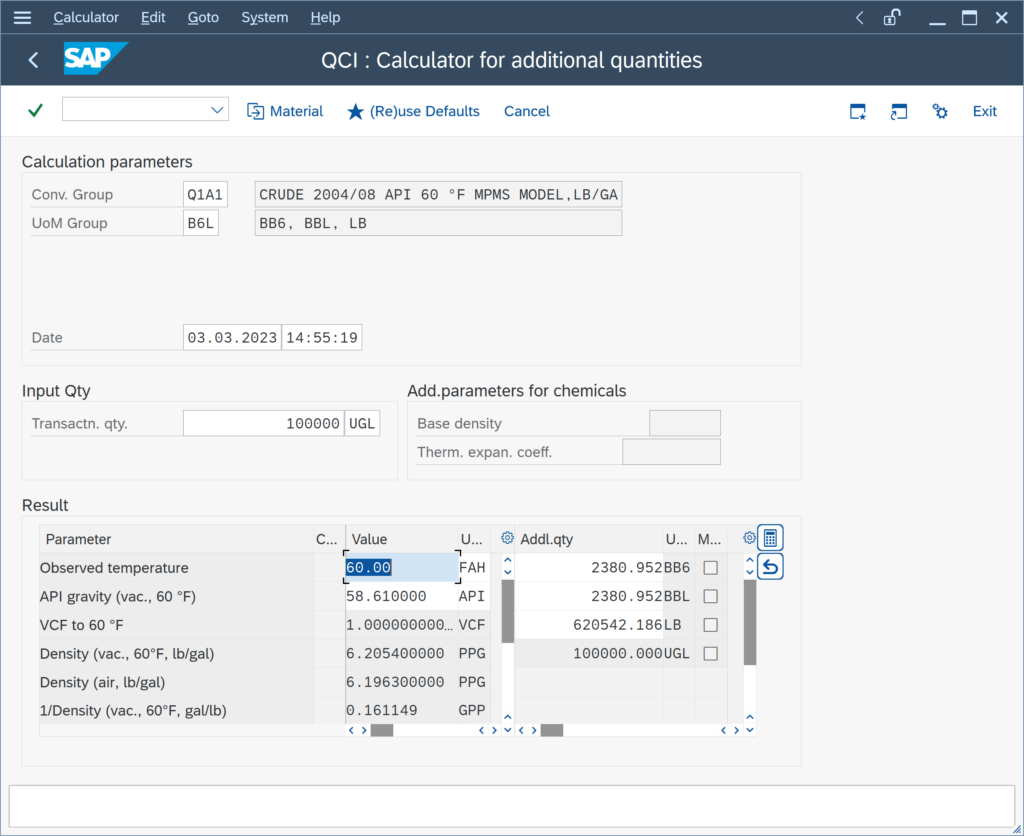This is due to the fact, that the density rounding function /QTYW/MQCI_ROUND_DENSITIES_UOM is processed after the quantity conversion model function /QTYW/MPMS_OBS_TO_ALT_QUAN: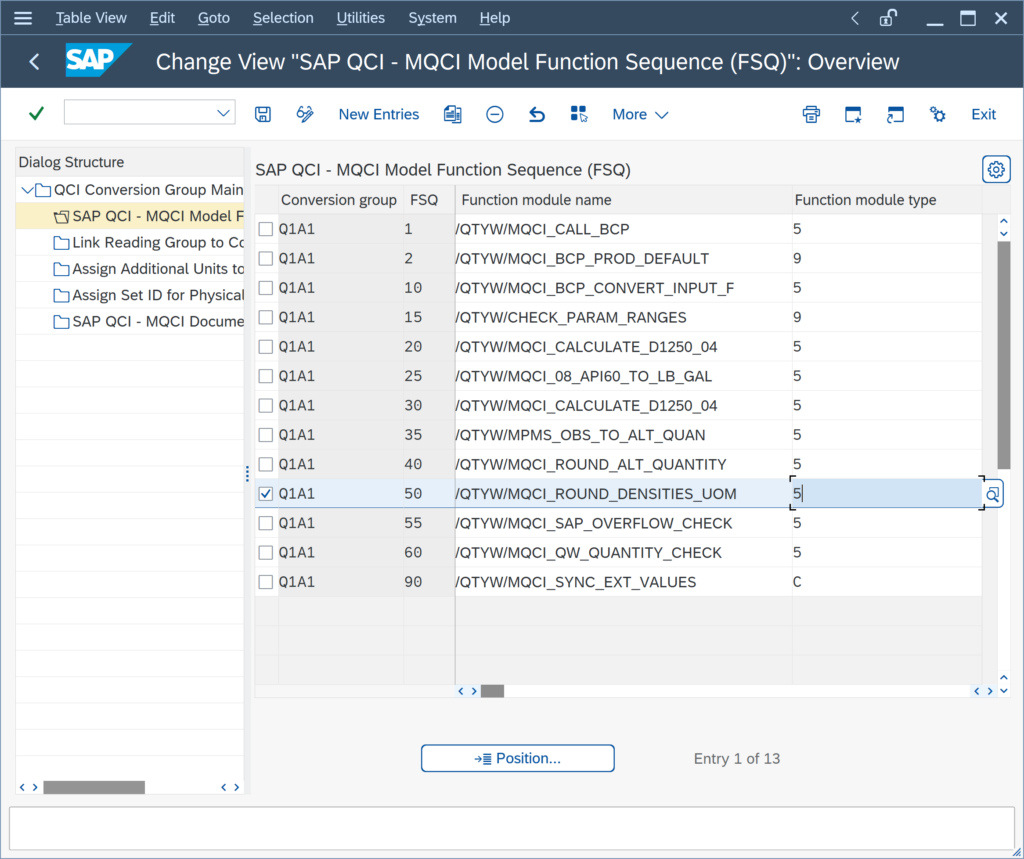If you position the rounding function before the quantity conversion model function: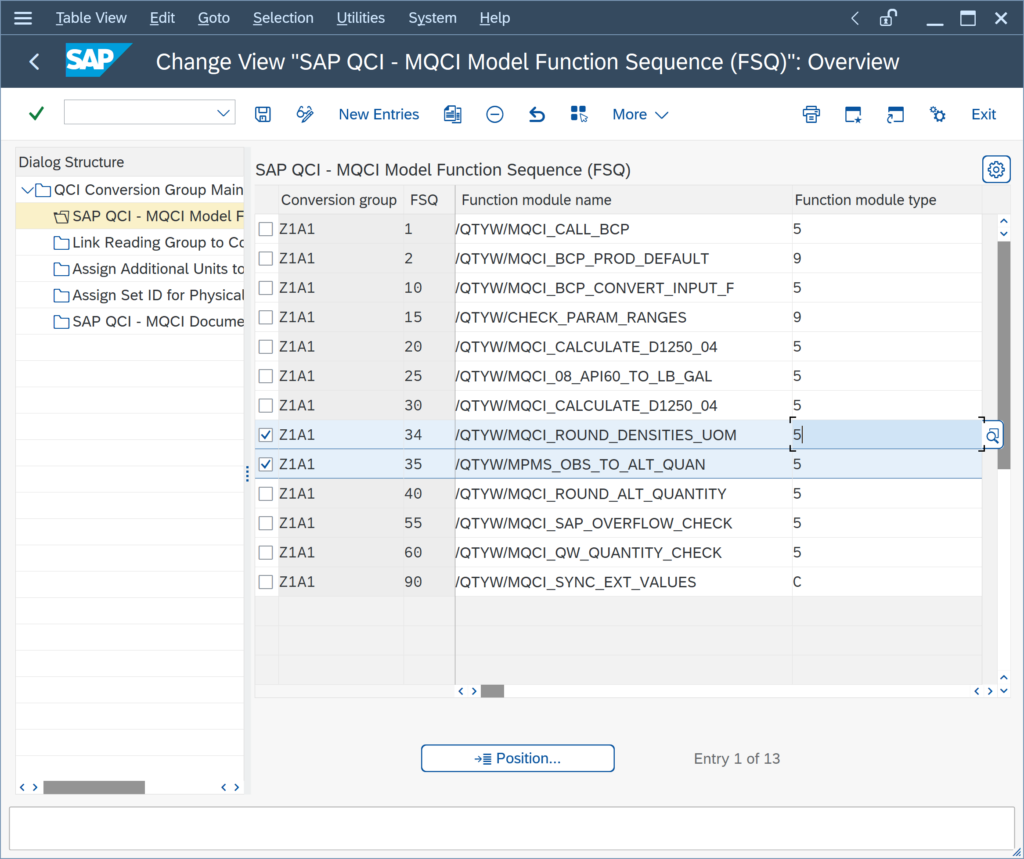the calculation is happening as expected: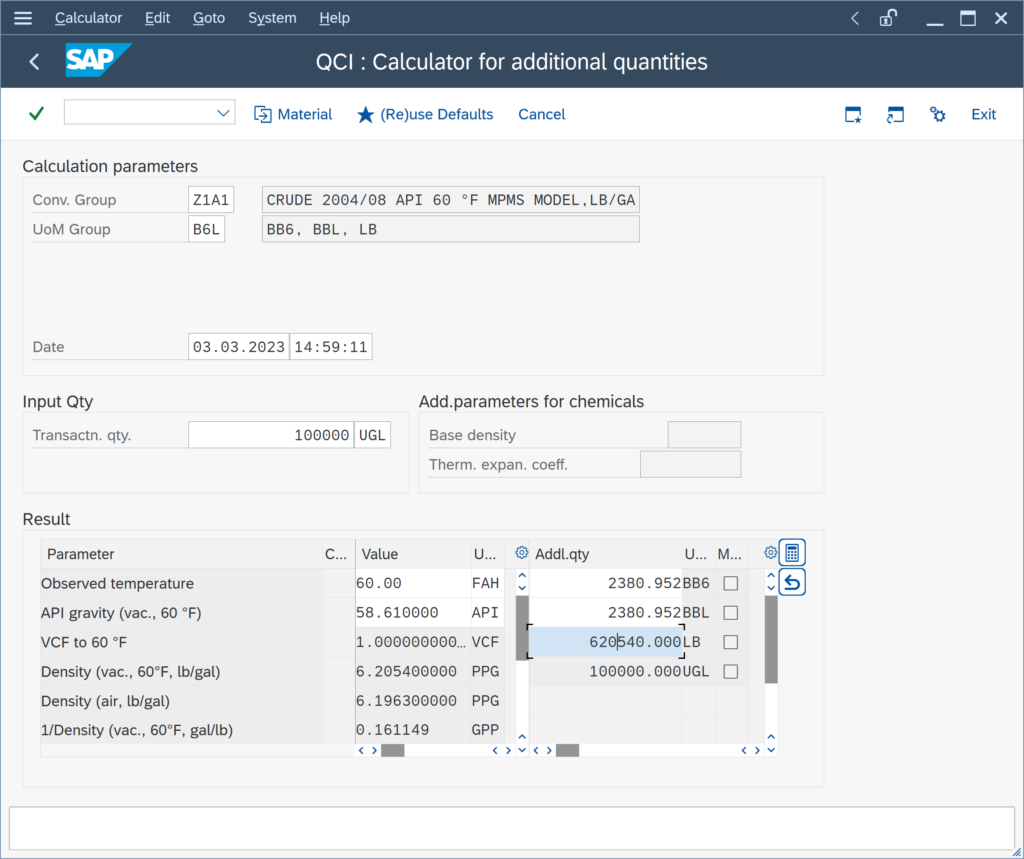The Q*** template conversion groups are configured to round the density values for reporting purposes,

### We do not require the hydrometer correction. Can we remove it from our conversion group configuration?

The hydrometer correction may only be required, if you enter an observed density that is determined with a glass hydrometer. If you utilize an ASTM D1250-04 implementation, you may simply remove the hydrometer function (and also remove the hydrometer indicator from the reading group):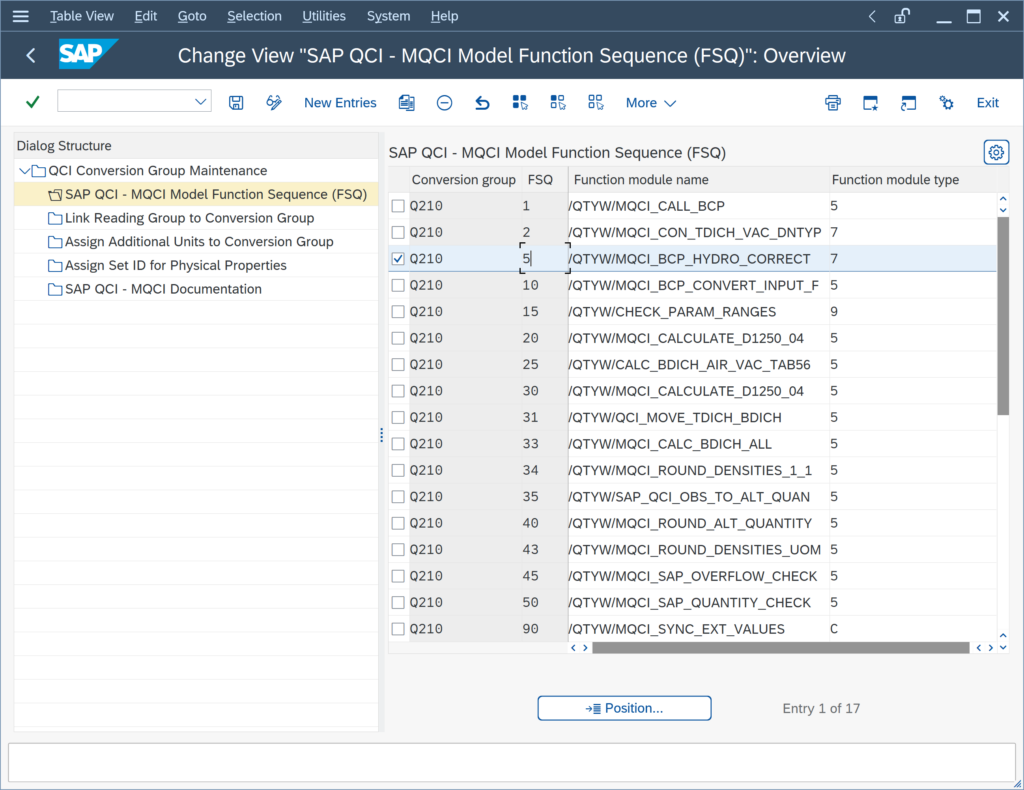ASTM D1250-80 implementations contain a built-in (by measurement standard definition) hydrometer correction logic, which requires that you actively turn the correction off if it is not required – read note 000026 for all important details.

### My client wants to use MQCI conversion groups, where UoM KG, LB, TO, STO ... are calculated as weights - is this possible?

Yes. The BCS configuration template provides example MQCI conversion groups that are configured such that UoM of SAP dimension ID MASS are calculated as weights (which is also the case for SAP QCI conversion groups if the air buoyancy indicator is set):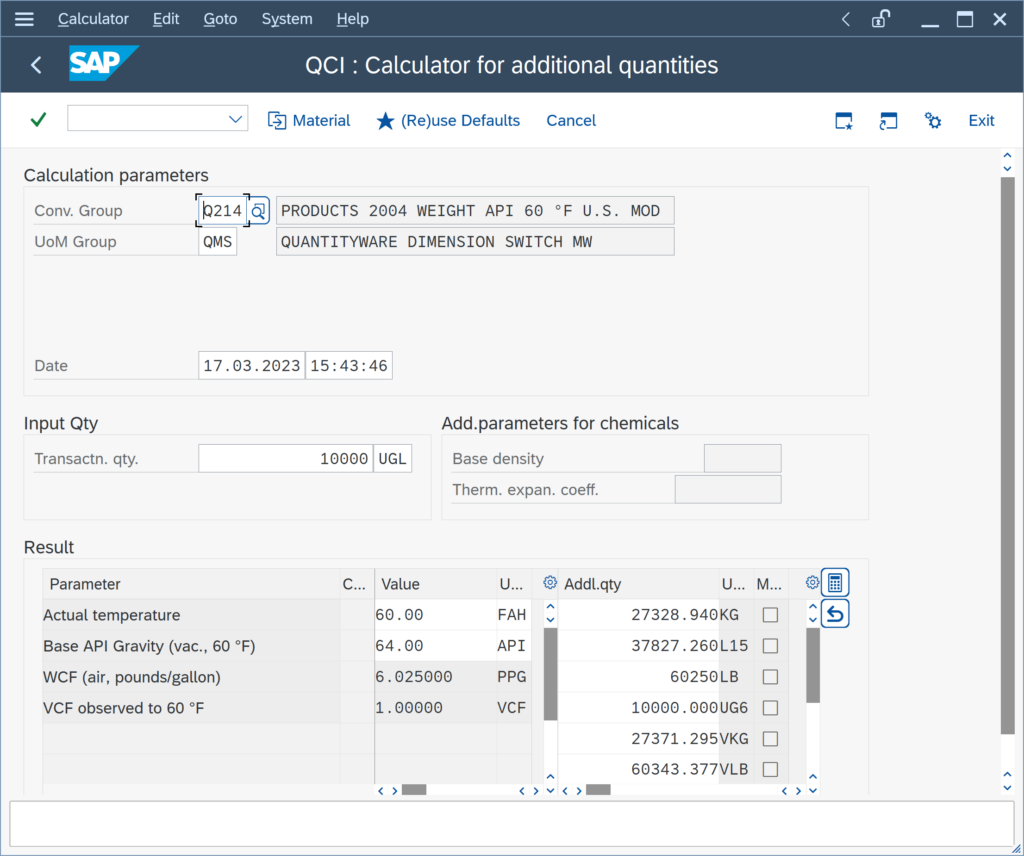The LB value is the weight of the 10 000 gallons of product. The configuration to achieve this requires 2 (plus 1) steps:

You assign the SAP Dimension IDs for weight (and mass) DIMID: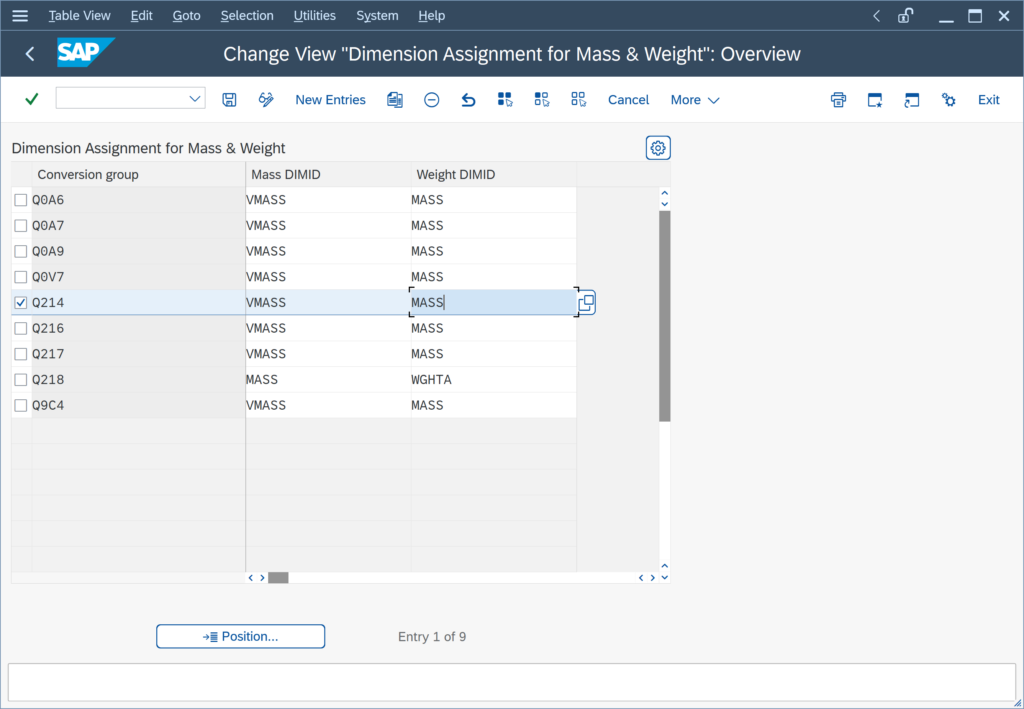You assign marker function /QTYW/MQCI_WEIGHT_MASS to the MQCI model function sequence: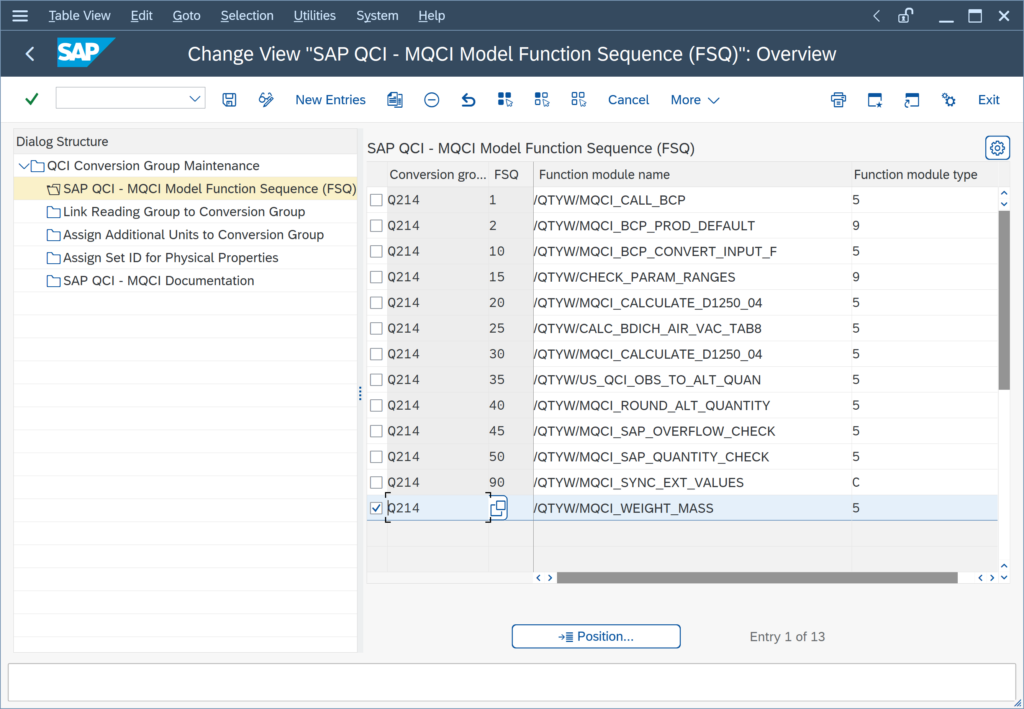Depending on the MQCI calculation model,

### My customer requires ASTM Table 1 UoM conversion factors. What are the configuration steps?

For MQCI conversion groups, you simply activate the ASTM Table 1 conversion factors and ASTM Table 1 version in the conversion group detail settings: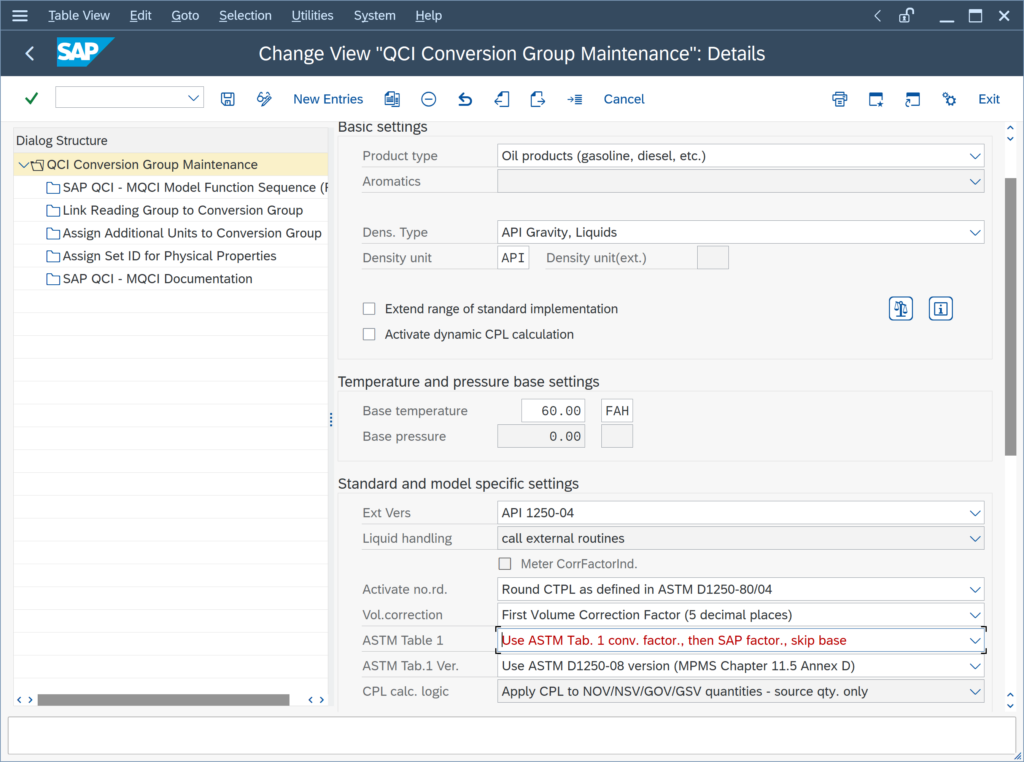With the QuantityWare BCS configuration templates, ASTM Table 1 conversion factors are delivered, which are accessible in your client 045 after activation of the relevant Business Configuration (BC) set. Read Note 000055 for all important additional configuration details.